• 当Y轴上的值差异性比较大的时候，如何进行坐标轴的截断，文档系统讲解了截断方法。
• 最全的条形图绘制方法总结，毕业论文绘图必须教程，方便快捷解决你的绘图困扰
• 29.R语言ggplot2——点图、折线图绘制方法教程.pdf
• ggplot2文档说明r语言ggplot2包的基本函数说明及实例
• 用于序列间的相关性矩阵计算，其中用到的其他语言包需自行下载，主题可自行调整
• R软件及Rstudio画图大全，ggplot2里面有很多关于R原件的画图代码及图形展示。希望对你有用。
• R语言ggplot2基础绘图案例 内含 1.条形图两张（详细代码及png图） 2.散点图三张（详细代码及png图） 3.折线图两张（详细代码及png图）
• 8. R语言ggplot2-主成分分析PCA加置信圈.pdf
• 32.R语言ggplot2和ggtext实现条形图加文本标注教程.pdf
• R语言ggplot包可以实现各种复杂的制图功能，本文以散点图为例，介绍ggplot2代码的使用方法。 首先，使用R内置数据attitude绘制complaints和learning的散点图。请注意ggplot2语法和R原生代码的区别。ggplot2采用...
• List item
R语言的ggplot包可以实现各种复杂的制图功能，本文以散点图为例，介绍ggplot2代码的使用方法。
首先，使用R内置数据attitude绘制complaints和learning的散点图。请注意ggplot2语法和R原生代码的区别。ggplot2采用图层模式，不同图层用“+”叠加。
rating complaints privileges learning raises critical advance
1     43         51         30       39     61       92      45
2     63         64         51       54     63       73      47
3     71         70         68       69     76       86      48

首先用ggplot()函数指定数据源，之后使用geom_point()函数绘制散点图，该函数使用mapping参数传入x和y所在的列。

ggplot(data = attitude) +
geom_point(mapping = aes(x = complaints, y = learning))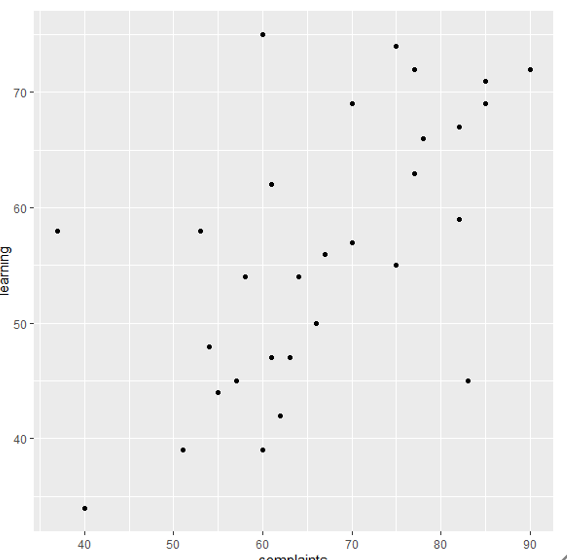那么，如何对散点进行分类呢？我们采用CO2数据。

Grouped Data: uptake ~ conc | Plant
Plant   Type  Treatment conc uptake
1   Qn1 Quebec nonchilled   95   16.0
2   Qn1 Quebec nonchilled  175   30.4
3   Qn1 Quebec nonchilled  250   34.8
4   Qn1 Quebec nonchilled  350   37.2
5   Qn1 Quebec nonchilled  500   35.3
6   Qn1 Quebec nonchilled  675   39.2

这次在aes中指定了color属性，设置为Plant列，这样可以对不同的Plant对应的散点应用不同的颜色。

ggplot(data = CO2) +
geom_point(mapping = aes(x = conc, y = uptake, color = Plant))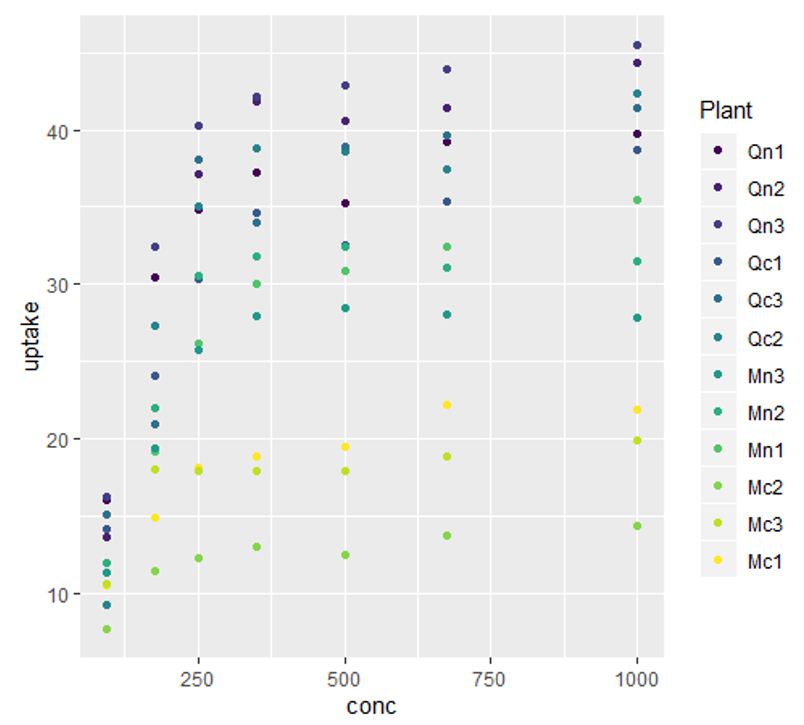同样，还可以指定size参数，使散点大小与某一参数相关。

ggplot(data = CO2) +
geom_point(mapping = aes(x = conc, y = uptake, color = Plant, size=conc))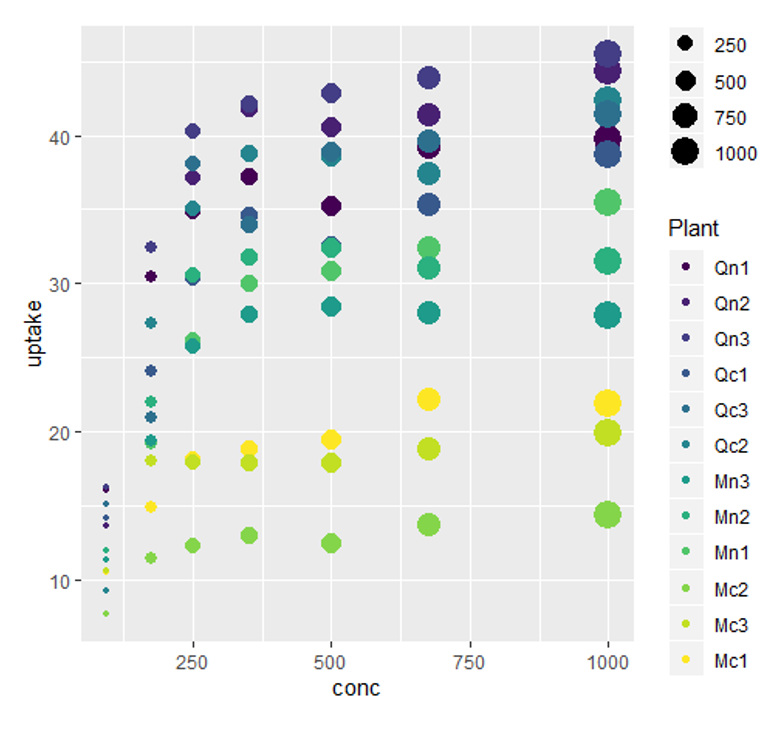指定shape参数，使散点的形状与某一参数相关。

ggplot(data = CO2) +
geom_point(mapping = aes(x = conc, y = uptake, color = Plant, size=conc, shape=Type))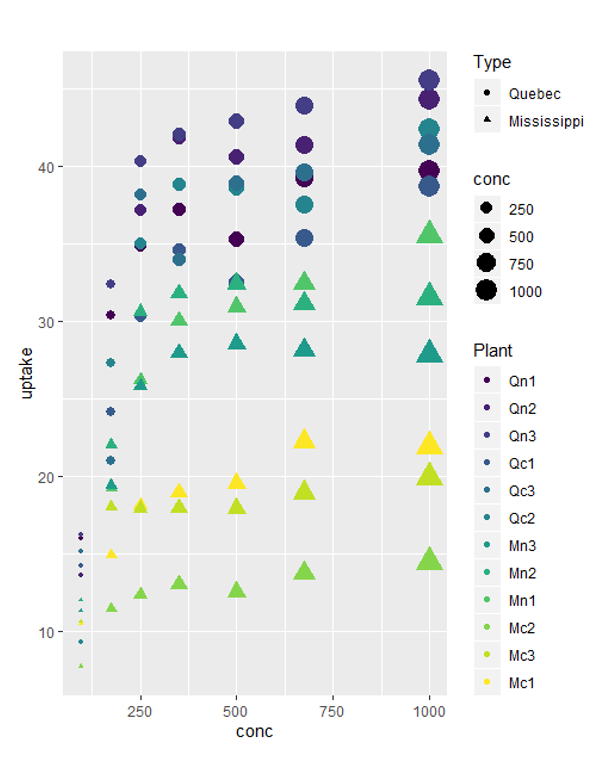下面使用iris数据展示ggplot2的更多功能。

Sepal.Length Sepal.Width Petal.Length Petal.Width Species
1          5.1         3.5          1.4         0.2  setosa
2          4.9         3.0          1.4         0.2  setosa
3          4.7         3.2          1.3         0.2  setosa

在mapping的aes参数中，还可以指定透明度alpha。

ggplot(data = iris) +
geom_point(mapping = aes(x = Sepal.Length, y = Sepal.Width, alpha=  Petal.Length , color = Species, shape= Species, size= Petal.Width))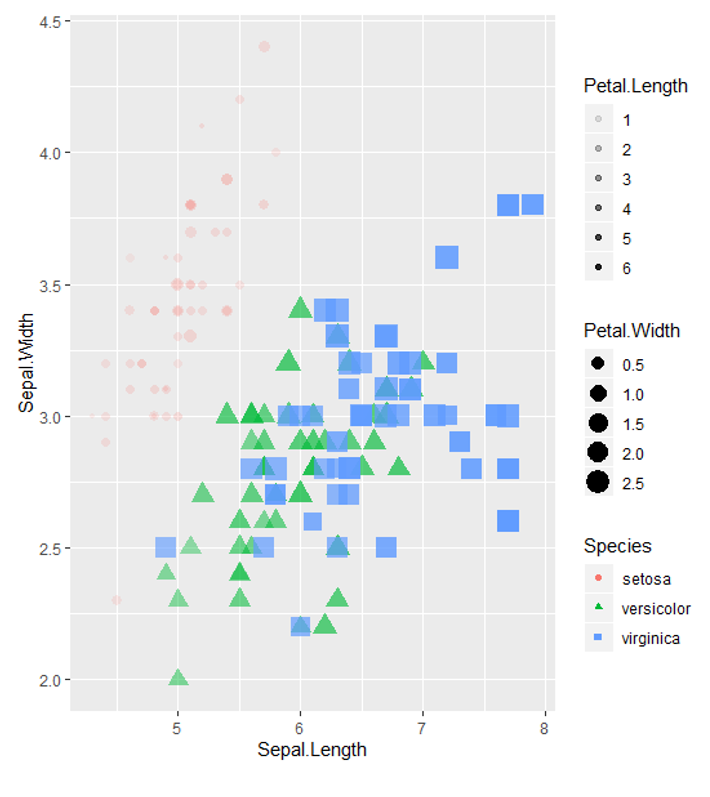以上这些图像都采取了默认的设置，如果我们想自定义散点的形状、颜色等参数时，该怎么办呢？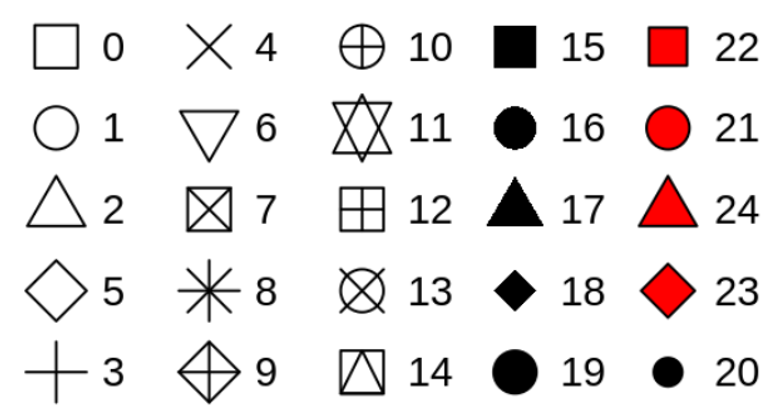引入color参数，可以自行设置颜色；使用shape参数可以自定义形状，各形状对应的序号如下。注意到color等参数是与mapping参数并列的。

ggplot(data = iris) +
geom_point(mapping = aes(x = Sepal.Length, y = Sepal.Width), color = "red",shape=11)

ggplot(data = iris) +
geom_point(mapping = aes(x = Sepal.Length, y = Sepal.Width, color= Species), color = c("#0FC62A","orange","#ABC"))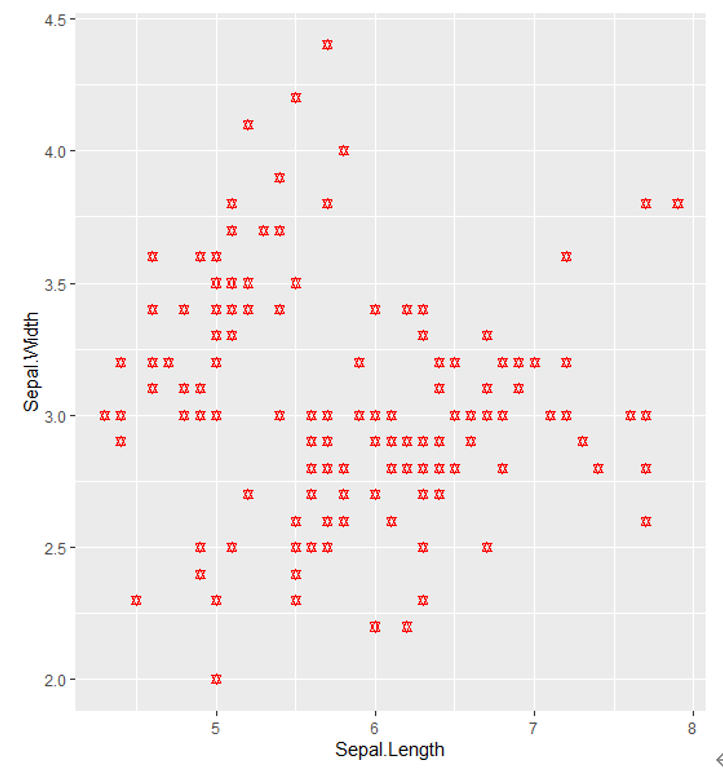2.3.3.2. 多图布局
如何将不同分类的变量绘制到不同的图上，实现多图布局呢？
在geom_point函数后用“+”连接facet_wrap()函数，其中首个参数为用于分类的变量前加“~”，nrow参数表示每行布局的图像数。

ggplot(data = iris) +
geom_point(mapping = aes(x = Sepal.Length, y = Sepal.Width, color = Species, shape= Species, size= Petal.Length)) +
facet_wrap(~ Species, nrow = 2)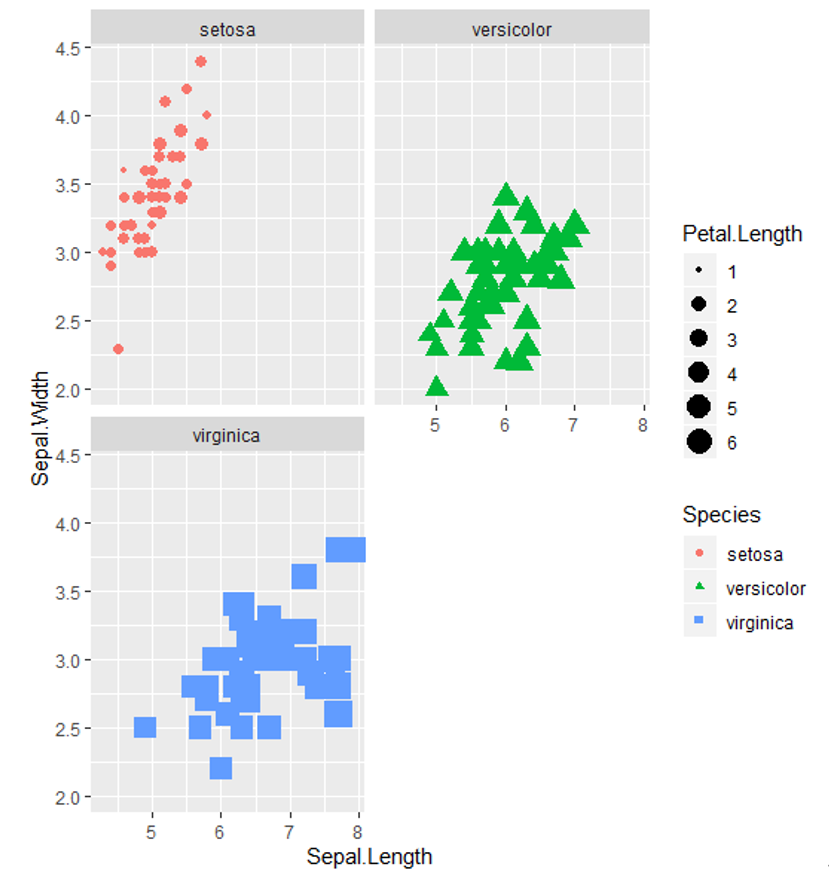如果需要用两个变量实现多图布局，可使用facet_grid()函数指定行列对应的变量，用“~”分隔。

ggplot(data = CO2) +
geom_point(mapping = aes(x = conc, y = uptake, color=Plant)) +
facet_grid(Type ~ Treatment)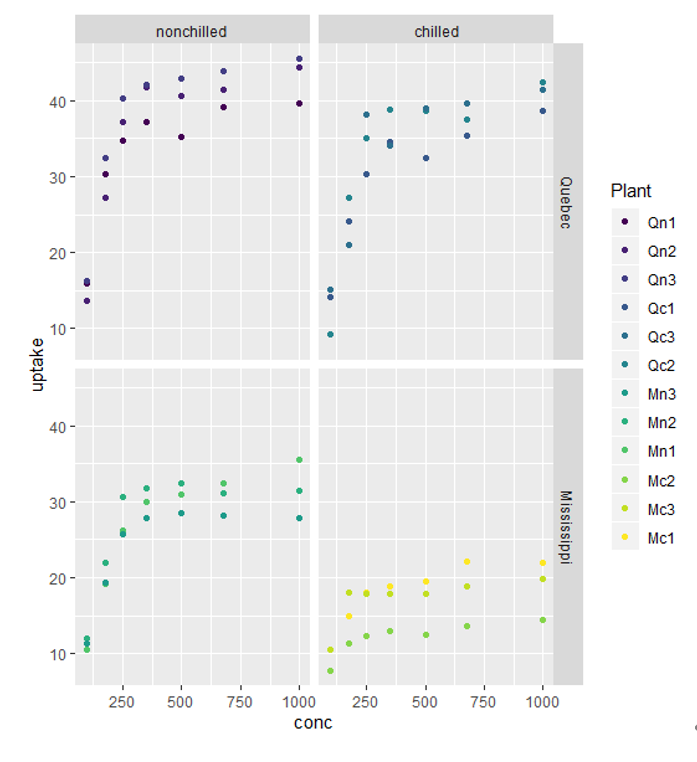若“~”前后的参数换为“.”，则只在列或行进行多图布局。

ggplot(data = CO2) +
geom_point(mapping = aes(x = conc, y = uptake, color=Plant)) +
facet_grid(Type ~ .)
ggplot(data = CO2) +
geom_point(mapping = aes(x = conc, y = uptake, color=Plant)) +
facet_grid(. ~ Treatment)

散点图常常会出现点重叠的情况，尤其是数据四舍五入后作图。通过调整参数position = “jitter”，可以避免这种网格化，为每个点添加少量随机噪声。因为没有两个点可能会接收到相同数量的随机噪声，所以这就使避免了散点堆积的情况。

ggplot(data = CO2) +
geom_point(mapping = aes(x = conc, y = uptake, color=Plant), , position = "jitter") +
facet_grid(Type ~ Treatment)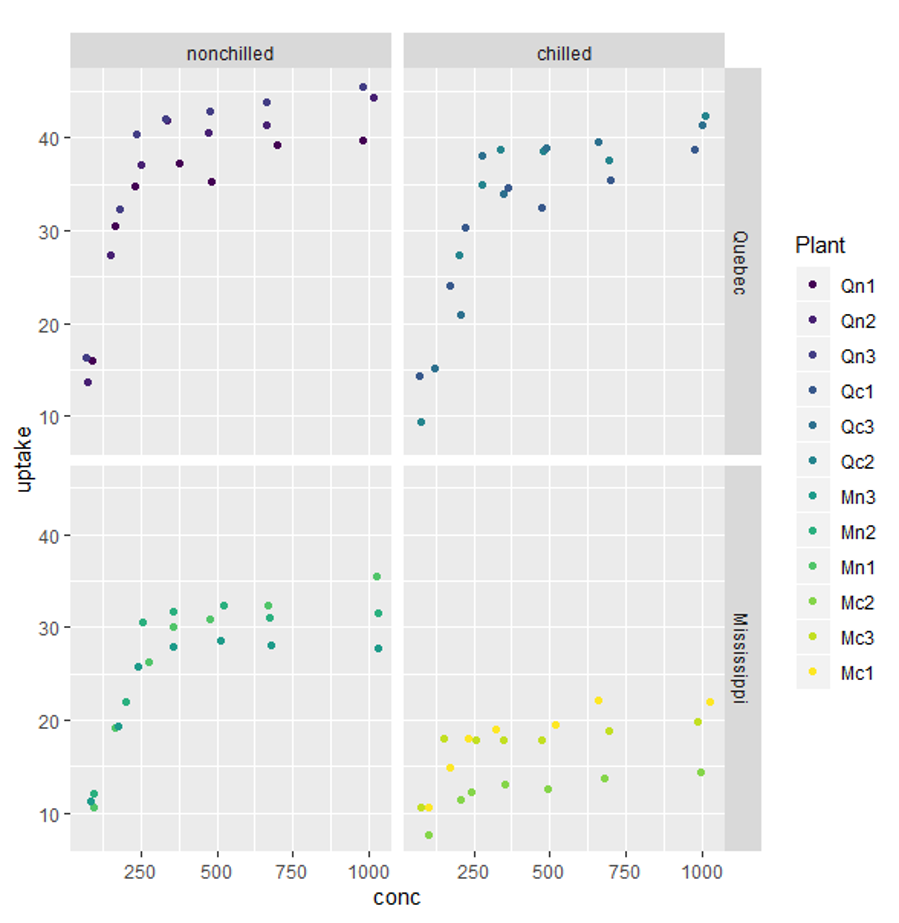展开全文r语言 ggplot2
• 9. R语言ggplot2绘图基础篇-柱状图加误差棒.pdf
• R语言ggplot2修改图例

千次阅读 2020-01-04 15:05:02
R语言修改图例。
序号操作代码
1修改图例名称labs(fill = “name”)
2隐藏/删除图例标题theme(legend.title = element_blank())
3修改图例顺序scale_color_discrete(limits = c(“a”, “d”, “c”))
4不加legendtheme(legend.position = “none”)
5修改legend位置theme(legend.position = “left”) #(right, top, bottom)
6修改尺寸大小theme(legend.text = element_text(colour = “red”, angle = 45, size = 10, hjust = 3, vjust = 3, face = “bold”))
7颜色修改及一致性geom_bar(position = “stack”, aes(order = desc(name)))
展开全文R语言 ggplot2
• 一、R语言的安装。 1.在搜索引擎中输入网址：R: The R Project for Statistical Computing (r-project.org)R: The R Project for Statistical Computing (r-project.org)，跳转到R语言官网。 R: The R Project for...

一、R语言的安装。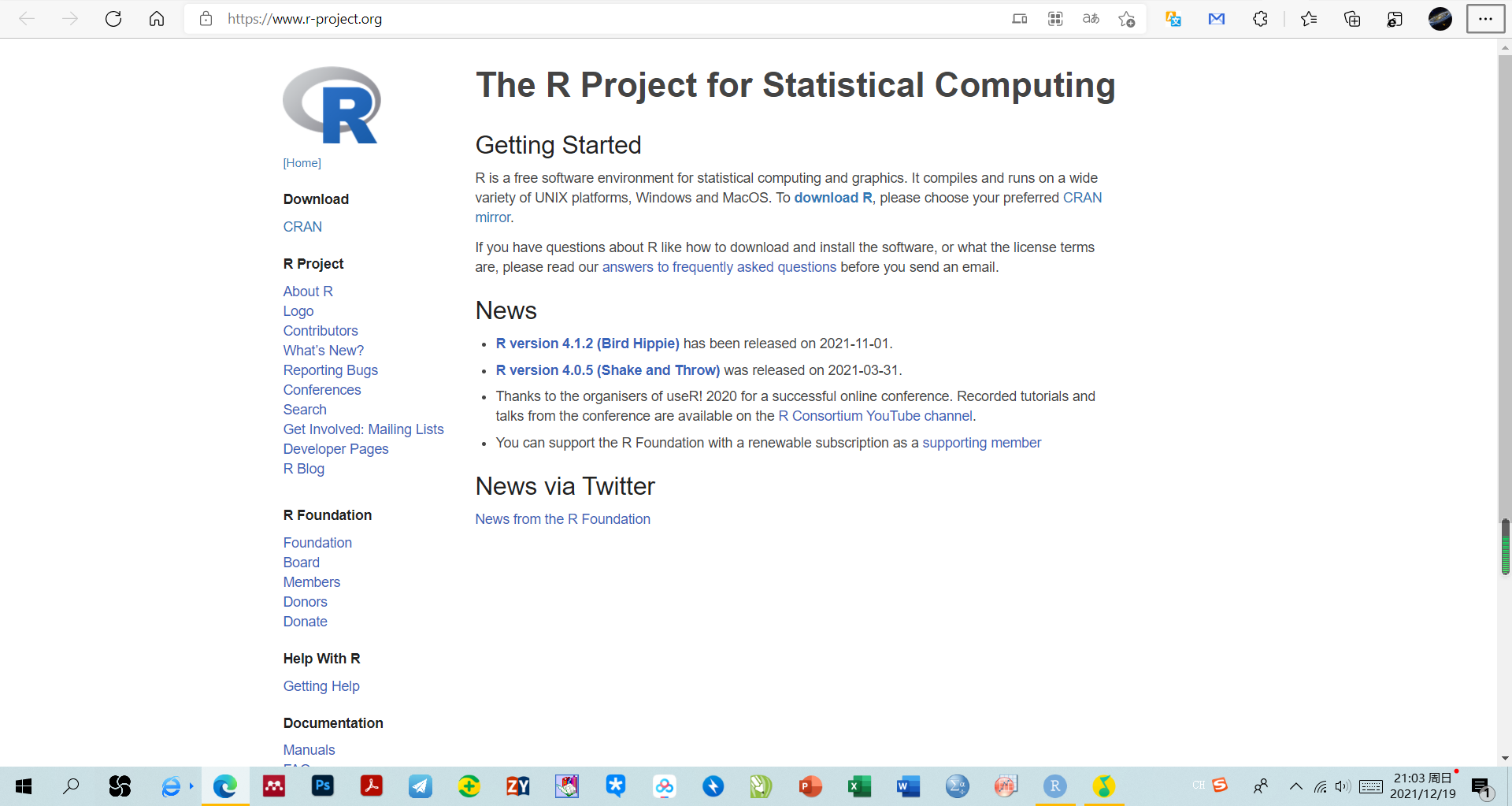R: The R Project for Statistical Computing (r-project.org)

2. 点击download R，跳转结果如下：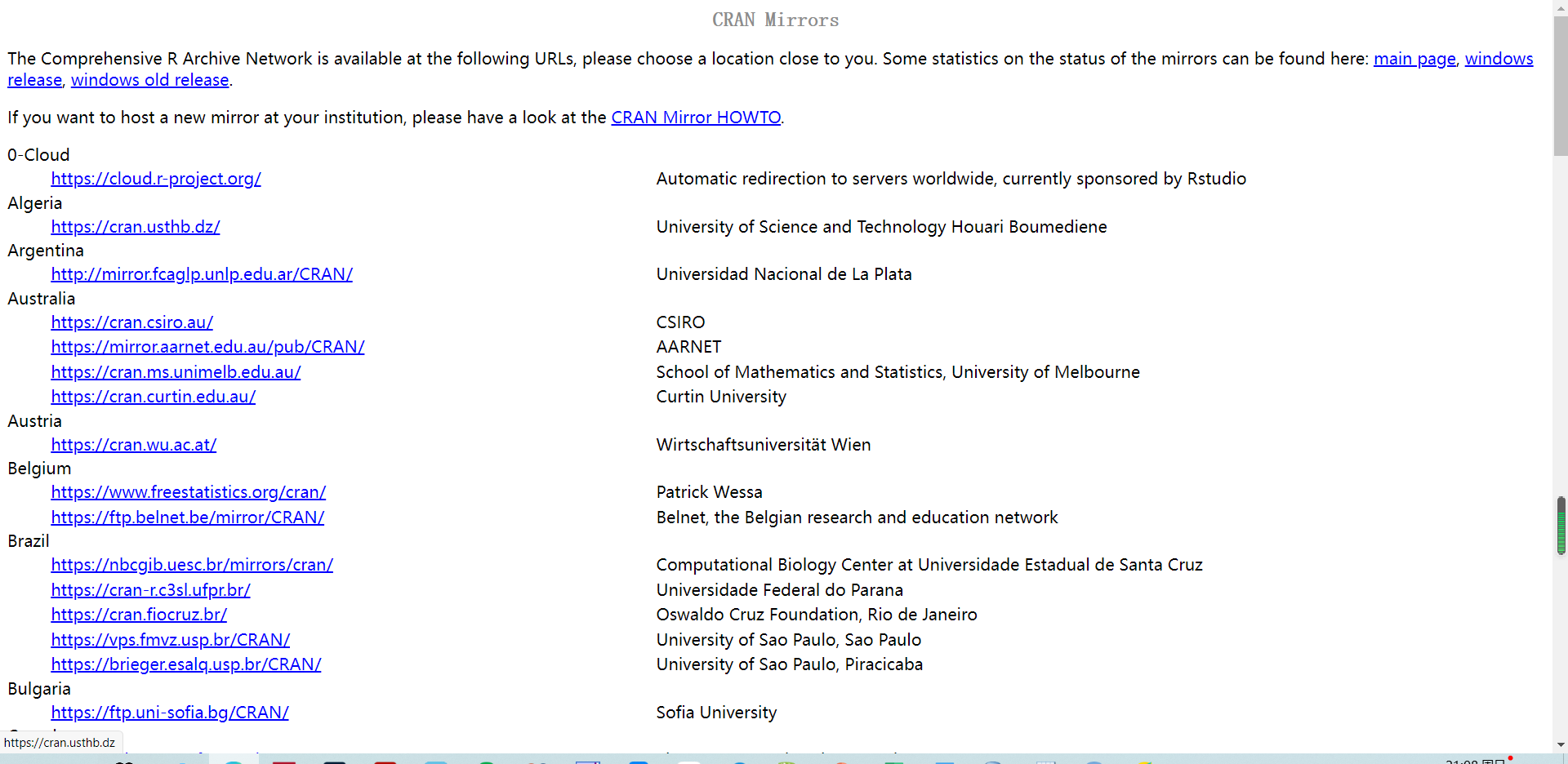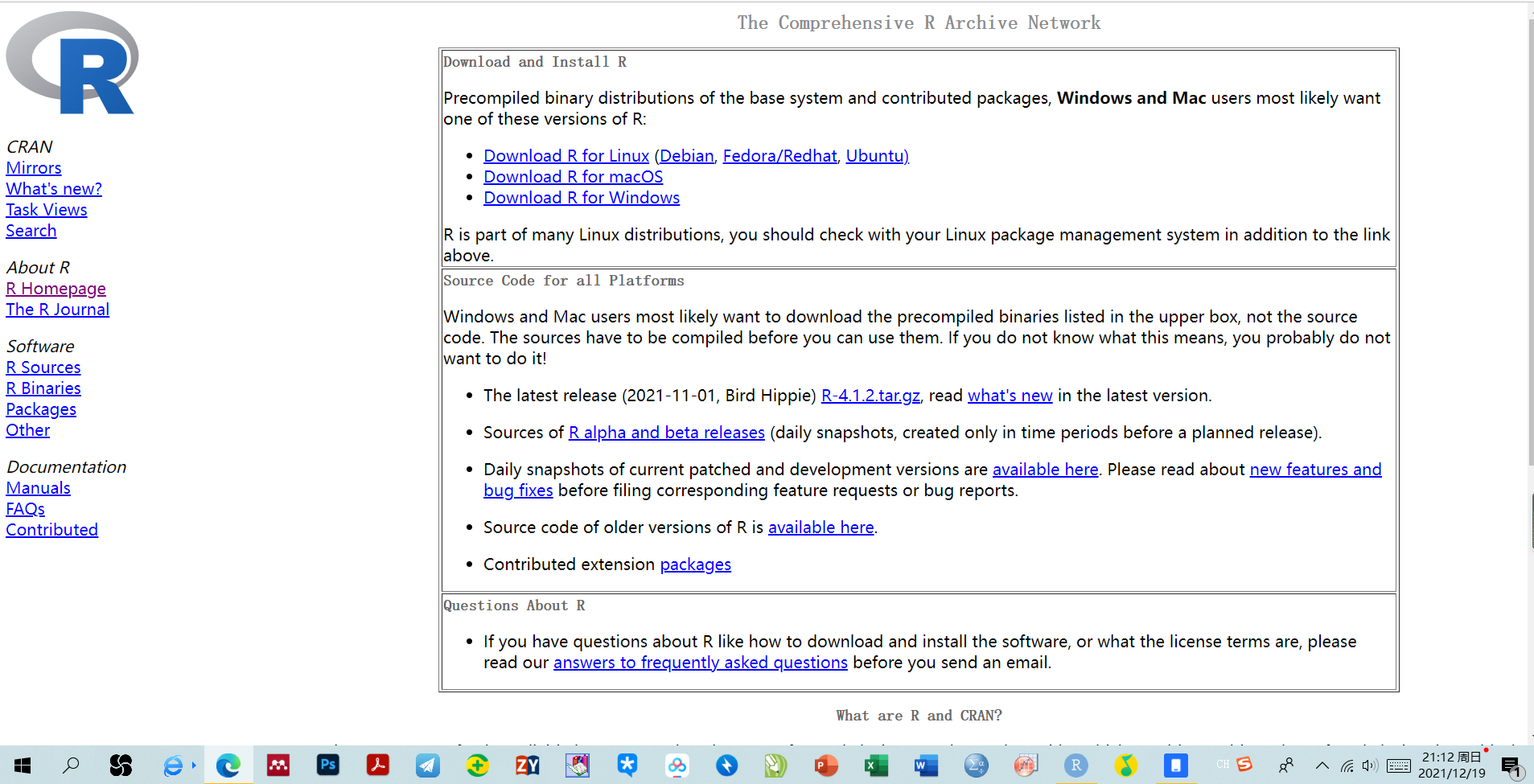4.点击后得到以下界面，点击install R for first time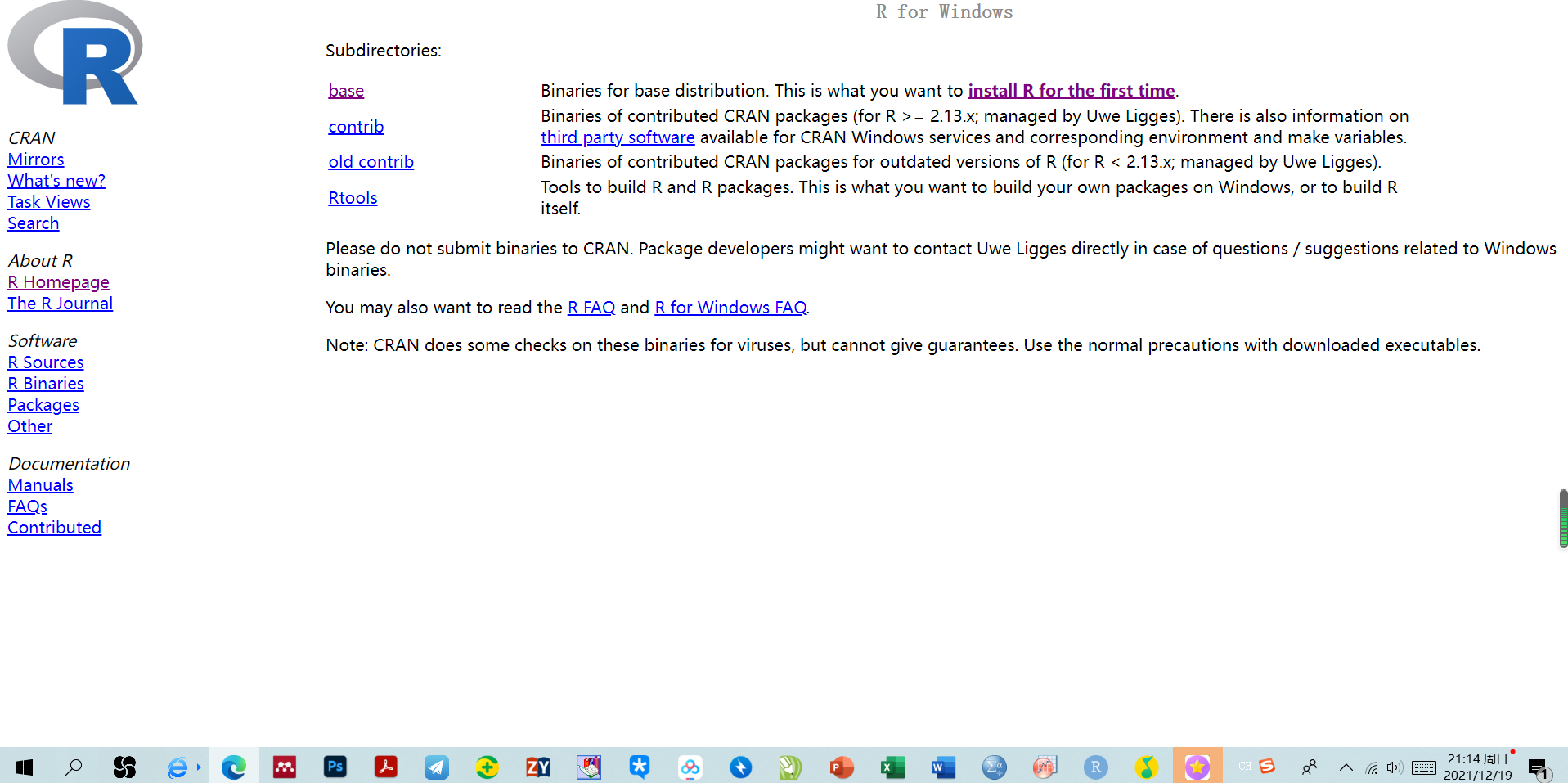5.点击后，选折下载最新版本的R。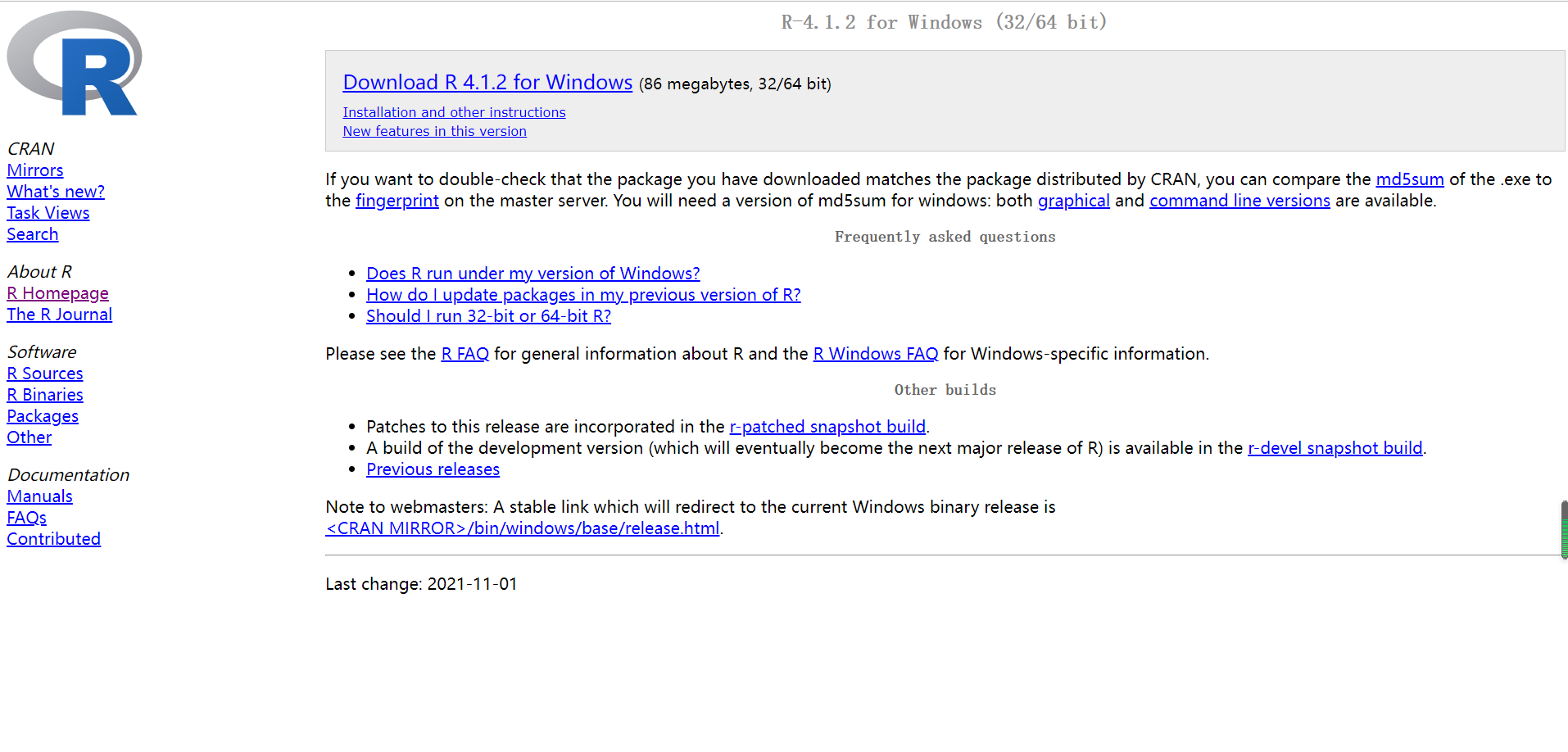二、RStudio的安装。

1.在搜索引擎输入网址RStudio | Open source & professional software for data science teams - RStudio，跳转到如下页面。2.点击Products，如下图，然后点击Rstudio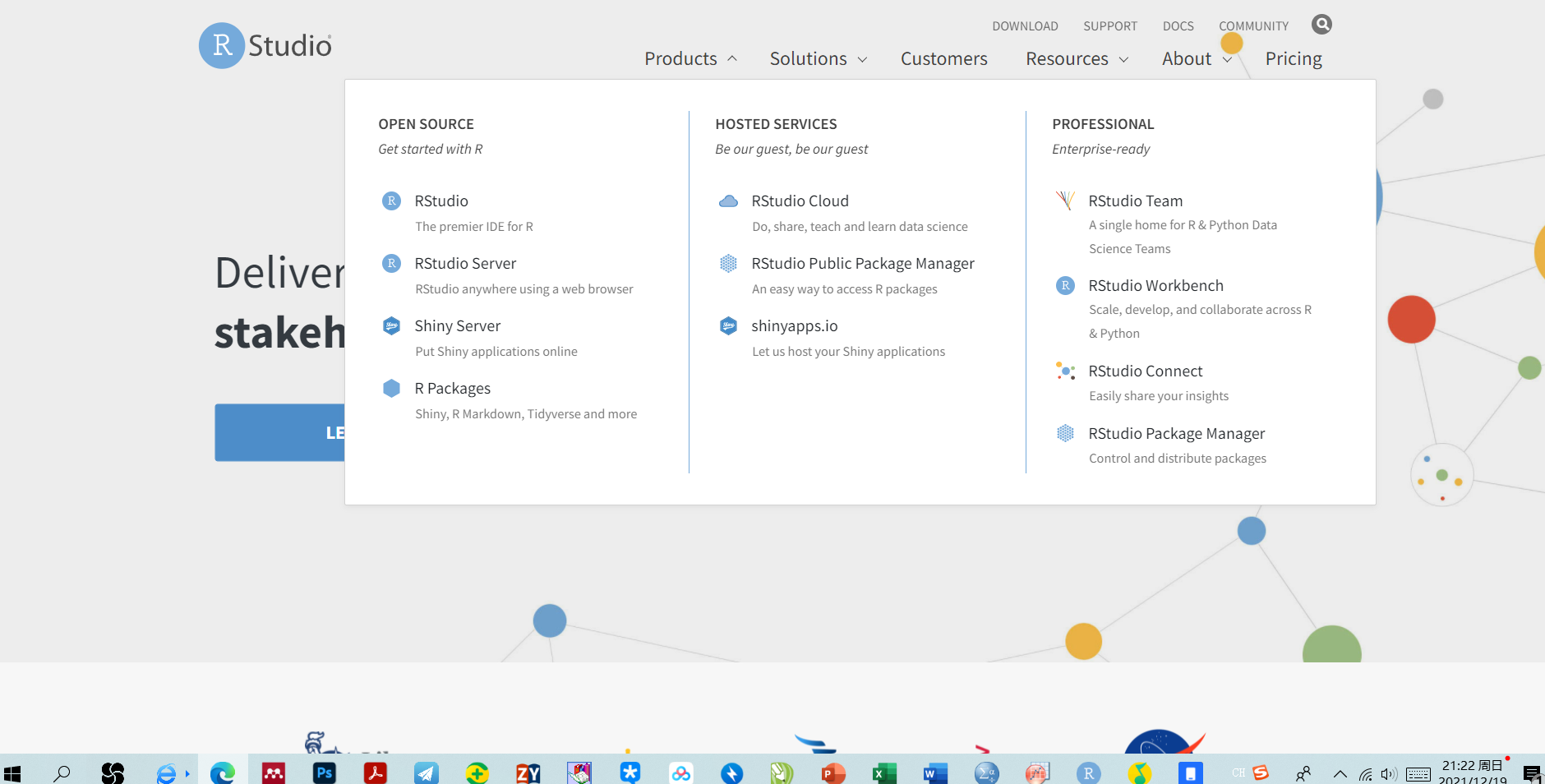3.跳转后，选择Rstudio Desktop，跳转下一页。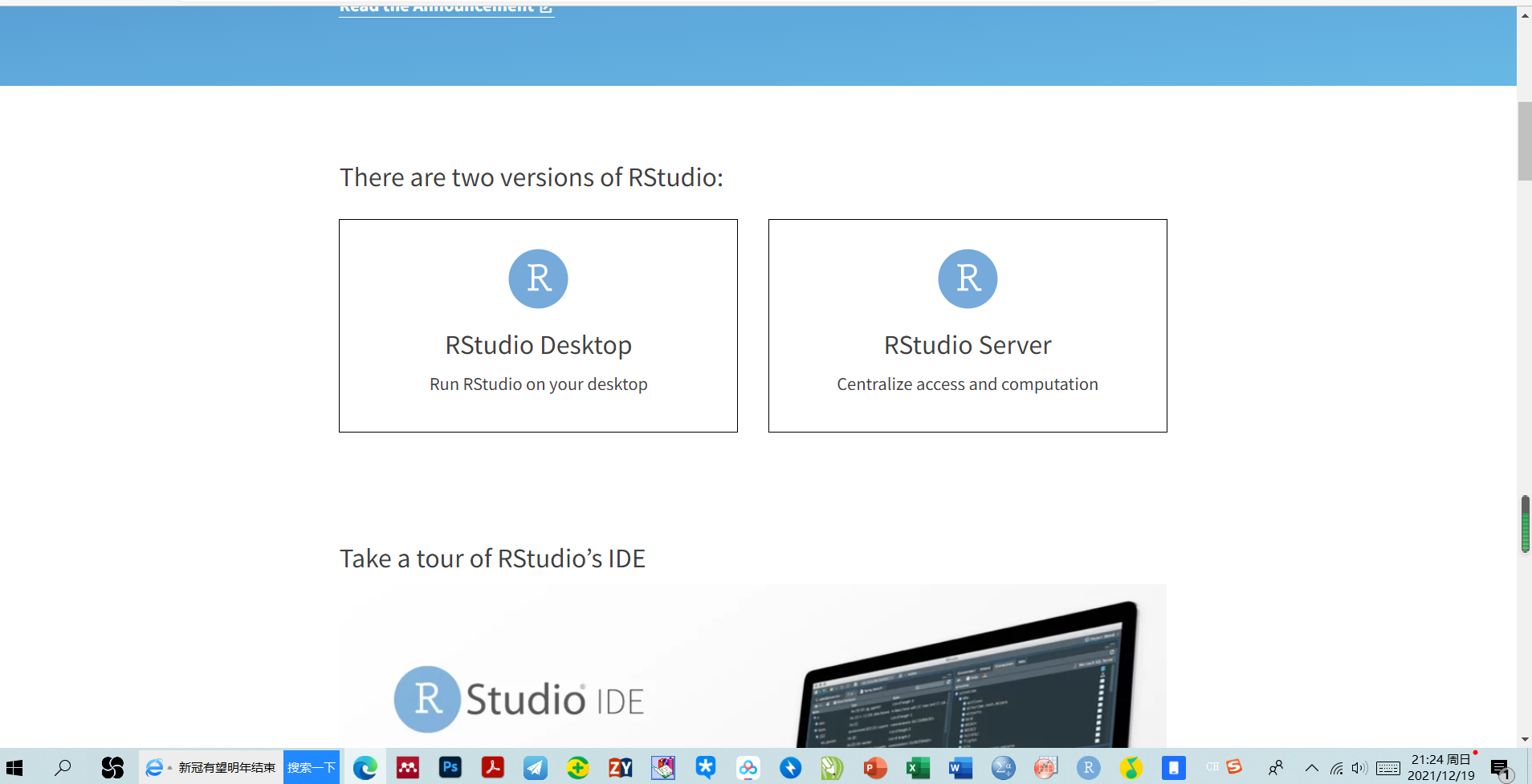4.点击DOWNLOAD RSTUDIO DESKTOP进行跳转。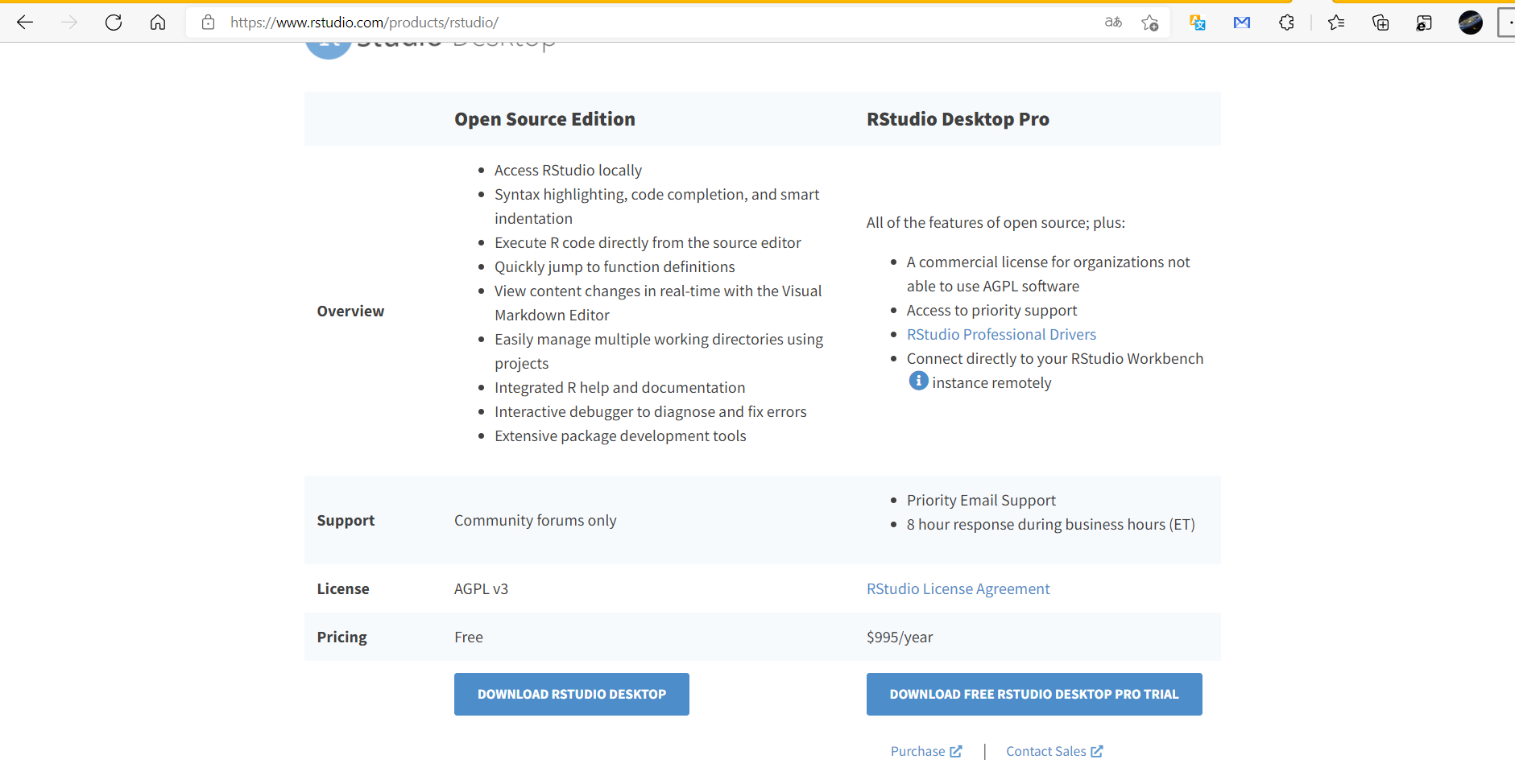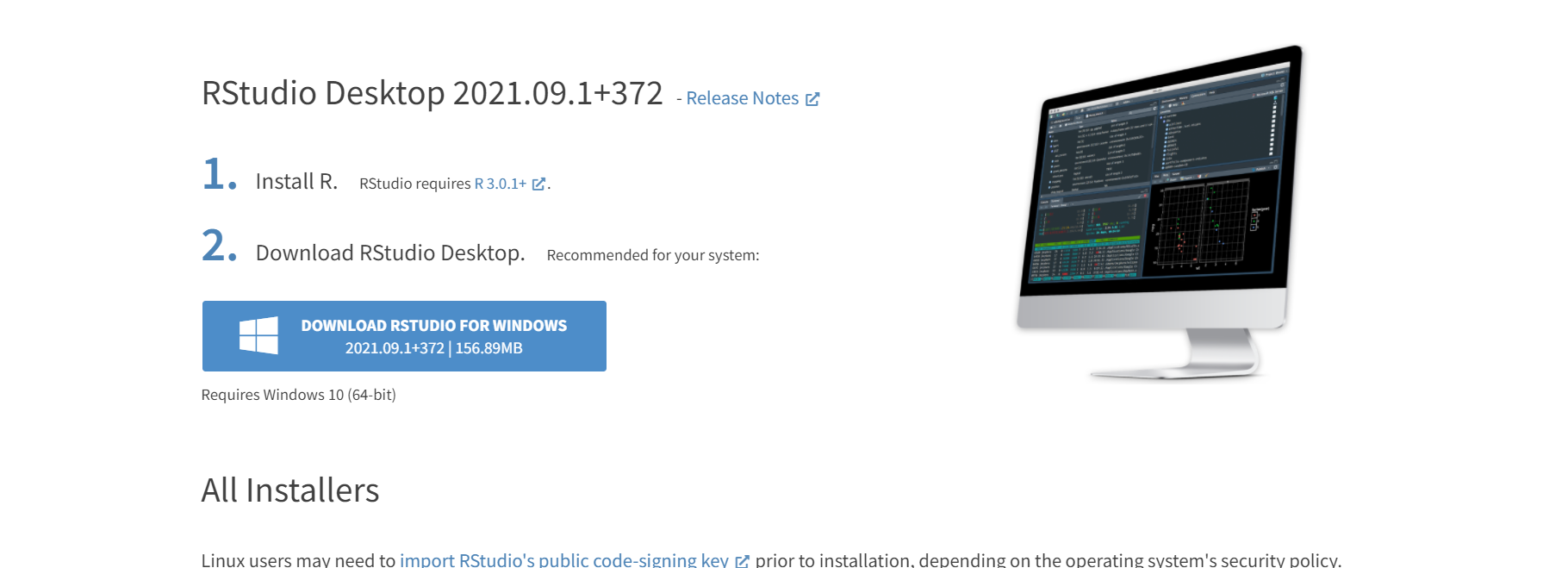三、R语言与RStudio下载成功后打开RStudio，如下图。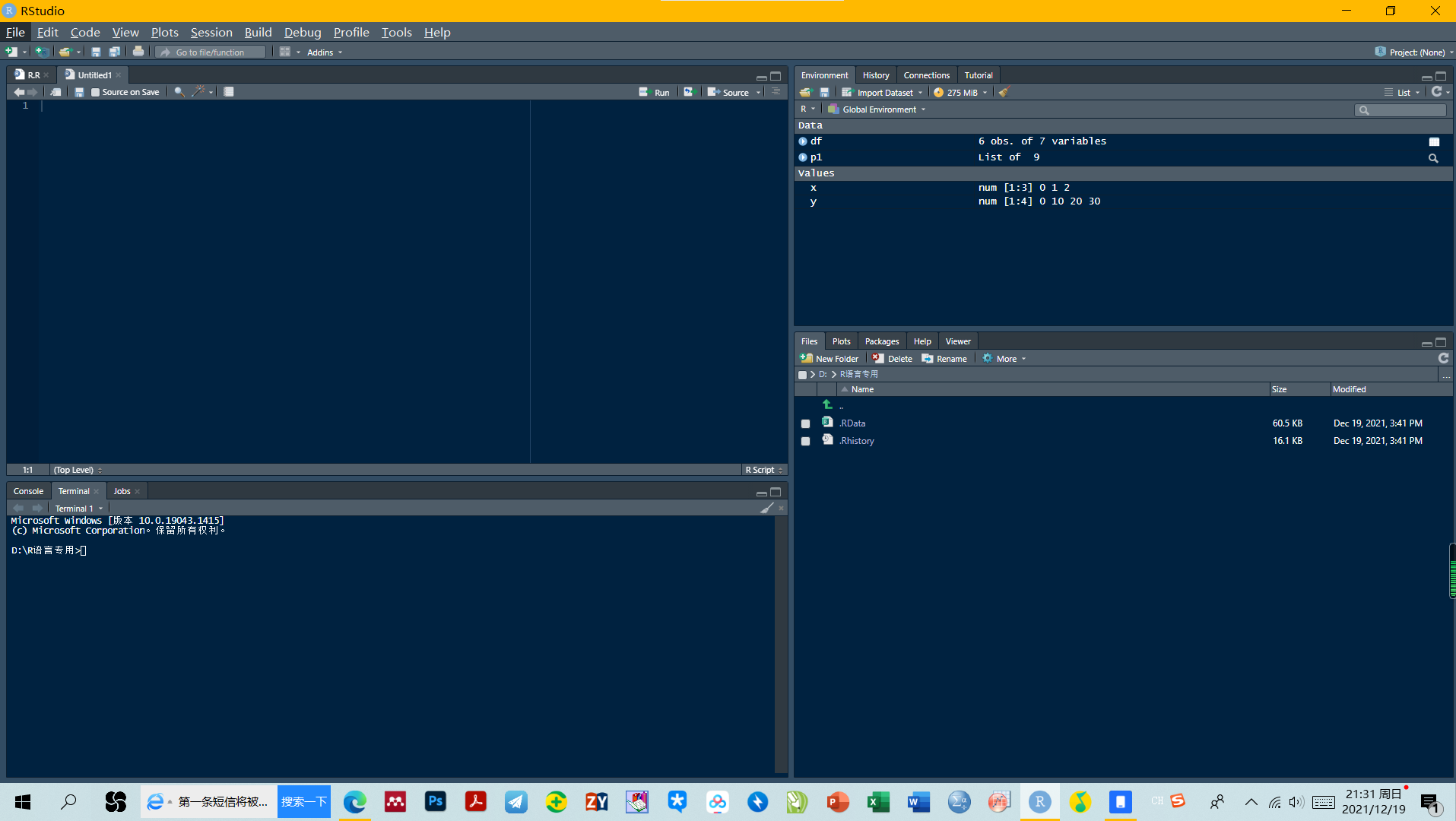四、ggplot2的下载与调用。

1.首次使用在代码框中输入：install.packages(“ggplot2”)，然后会自动下载成功。（注意同时下载时R语言和RStudio均选最新版本，否则会造成R语言和RStudio不兼容而下载失败

2.选中install.packages(“ggplot2”)，点击run运行，ggplot2安装成功。

3.以后使用ggplot2之前输入代码library（“ggplot2”）即可，选中library(“ggplot2”)点击run运行。

展开全文r语言 开发语言
• <code class="language-R">p9 <- ggplot(PT9, aes(x=温度, y=频率,group=1,colour=体感热度)) + geom_point(size=2,shape=21) + #加点 geom_smooth(method = "lm"...r语言
• R语言绘制箱线图箱线图(又称盒须图)是常见的统计图形之一，通过绘制连续型变量的五数总括，即最小值、下四分位数(第25百分位数)、中位数(第50百分位数)、上四分位数(第75百分位数)以及最大值，描述了连续型变量的...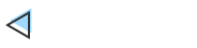R语言绘制箱线图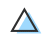箱线图(又称盒须图)是常见的统计图形之一，通过绘制连续型变量的五数总括，即最小值、下四分位数(第25百分位数)、中位数(第50百分位数)、上四分位数(第75百分位数)以及最大值，描述了连续型变量的分布。箱线图能够显示出可能为离群点(范围±1.5×IQR以外的值，IQR表示四分位距，即上四分位数与下四分位数的差值)的观测。

默认情况下，两条须的延伸极限不会超过盒型各端加1.5倍四分位距的范围。此范围以外的值以点(即离群点)来表示。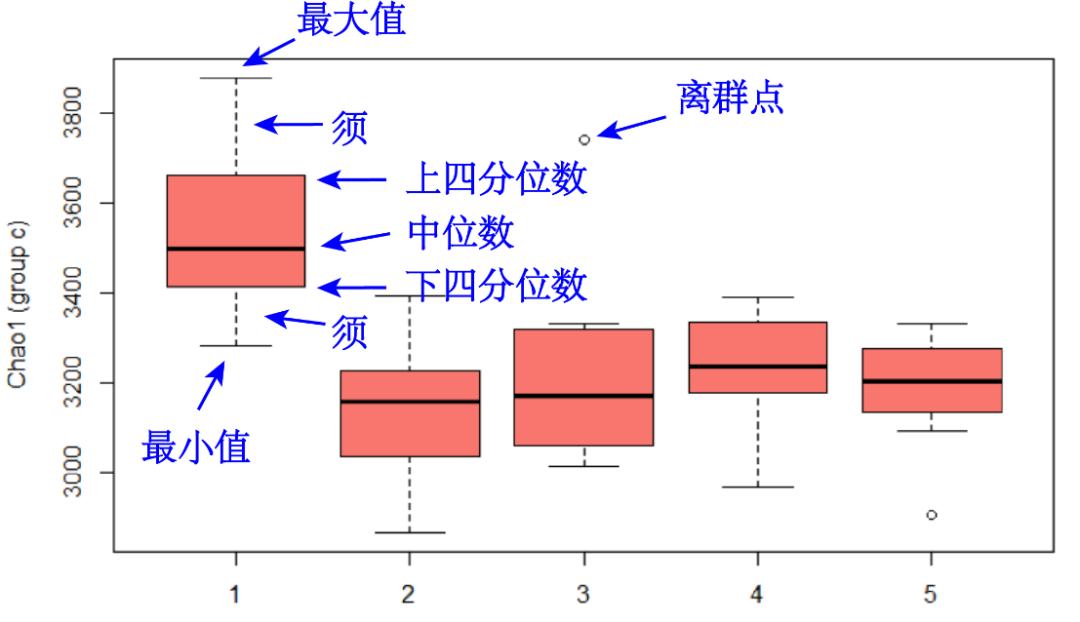本篇通过分享R语言绘制箱线图的几种常见样式的简单示例，以帮助入门的同学们掌握常规的作图技巧。

作图数据的网盘链接(提取码pmbr)：

https://pan.baidu.com/s/1WZ2UzXPGw9M448v-fms6RA

网盘附件“alpha.csv”为某16S细菌群落测序所获得的部分alpha多样性指数数据。其第一列为样本名称；observed_species、shannon、chao1和PD_whole_tree为四种类型的alpha多样性指数，其中的数值代表了各样本中该alpha多样性指数的数值；group1和group2为样本所对应的分组信息。

本篇我们将通过绘制箱线图，展示alpha多样性指数在各分组中的分布概况。

R语言绘制箱线图的简单示例

我们首先将作图数据读到R中，并分别从中挑选部分数据，便于后续绘制不同的样式。

#读取数据
library(reshape2)
alpha alpha$group2 alpha1 alpha2 alpha3 boxplot()箱线图 R启动时默认加载了boxplot()命令，可用于绘制箱线图。我们使用boxplot()绘制箱线图展示chao1指数在c分组中的分布，如下示例。 ##boxplot() 箱线图，详情使用 ?boxplot 查看帮助 par(mfrow = c(1, 2)) #常规样式 boxplot(value~group2, data = alpha3, col = '#f8766d', ylab = 'Chao1 (group c)') #根据数据分布，添加凹槽 boxplot(value~group2, data = alpha3, col = '#f8766d', notch = TRUE, varwidth = TRUE, ylab = 'Chao1 (group c)')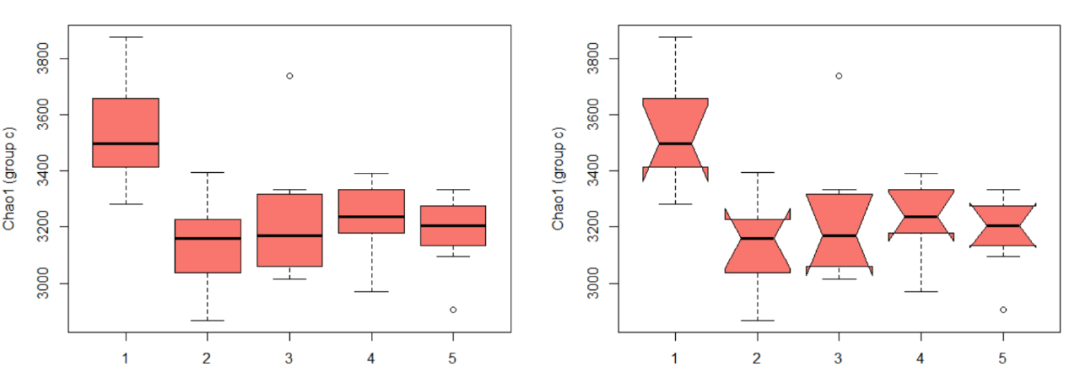ggplot2绘制箱线图 ggplot2功能强大自然不必多说，以下继续展示使用ggplot2绘制箱线图的示例。 ##ggplot2 library(ggplot2) #单变量箱线图 ggplot(alpha3, aes(x = group2, y = value)) + geom_boxplot(outlier.size = 1, fill = '#f8766d') + theme(panel.grid = element_blank(), panel.background = element_rect(fill = 'transparent', color = 'black')) + labs(x = '', y = 'Chao1')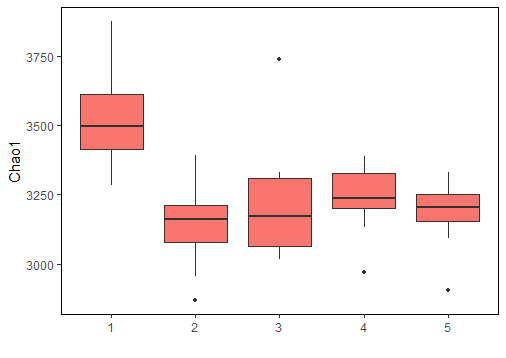#将各数据值以抖动散点的方式添加在箱线图中，同时绘制凹槽 ggplot(alpha3, aes(x = group2, y = value, fill = group1)) + geom_boxplot(fill = '#f8766d', notch = TRUE) + geom_jitter(color = 'red', show.legend = FALSE) + theme(panel.grid = element_blank(), panel.background = element_rect(fill = 'transparent', color = 'black')) + labs(x = '', y = 'Chao1 (group c)')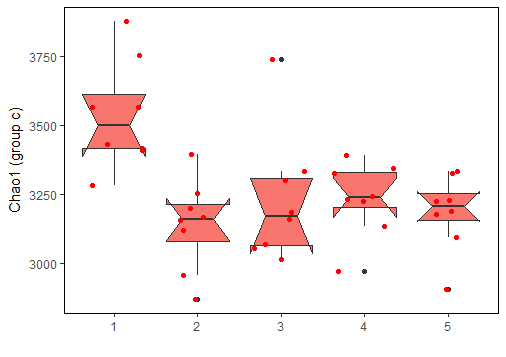#存在多分组时，多组分开展示的箱线图 ggplot(alpha2, aes(x = group2, y = value, fill = group1)) + geom_boxplot(outlier.size = 1) + theme(panel.grid = element_blank(), panel.background = element_rect(fill = 'transparent', color = 'black'), legend.title = element_blank(), legend.key = element_blank()) + labs(x = '', y = 'Chao1')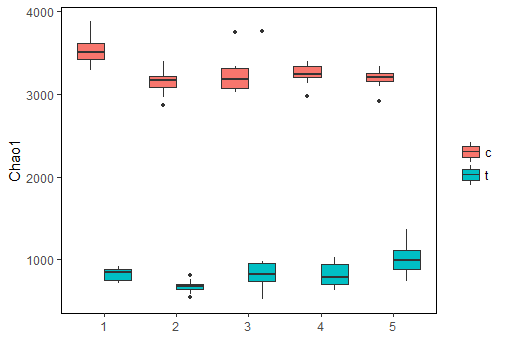#多变量情况，添加分面的箱线图 ggplot(alpha1, aes(x = group2, y = value, fill = group1)) + geom_boxplot(outlier.size = 0.5, size = 0.5) + facet_wrap(~variable, 2, scales = 'free') + theme(panel.grid = element_blank(), panel.background = element_rect(fill = 'transparent', color = 'black'), legend.title = element_blank(), legend.key = element_blank()) + labs(x = '', y = 'Chao1')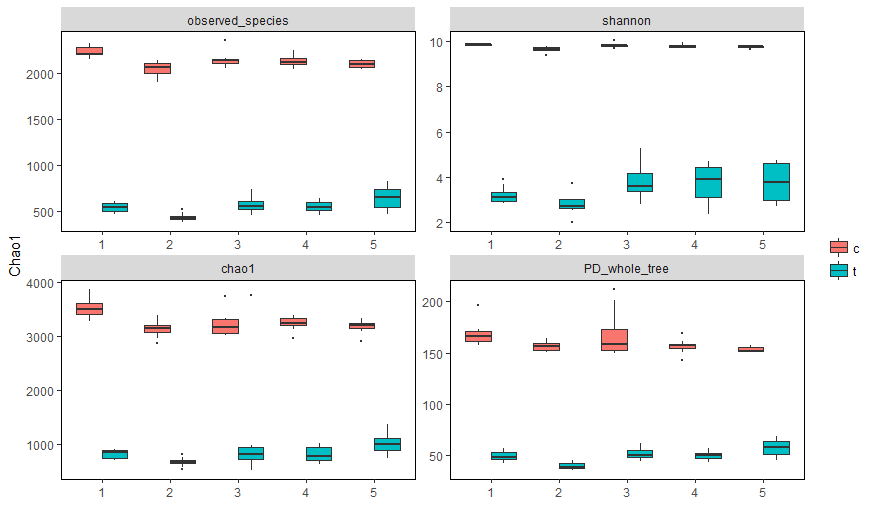#带显著性标记“*”的箱线图 #先绘制箱线图主体 p geom_boxplot(outlier.size = 1) + theme(panel.grid = element_blank(), panel.background = element_rect(fill = 'transparent', color = 'black'), legend.title = element_blank(), legend.key = element_blank()) + labs(x = '', y = 'Chao1') #再手动添加显著性标记 #注意，这里的显著性是提前已经计算好的，我们通过手动输入进来 #本篇只关注作图，不涉及统计分析 library(doBy) alpha2_stat names(alpha2_stat) alpha2_stat$group1 alpha2_stat$sig p + geom_text(data = alpha2_stat, aes(label = sig), vjust = -0.3) + annotate('text', x = alpha2_stat$group2, y = alpha2_stat$value, label = '———', vjust = -0.3)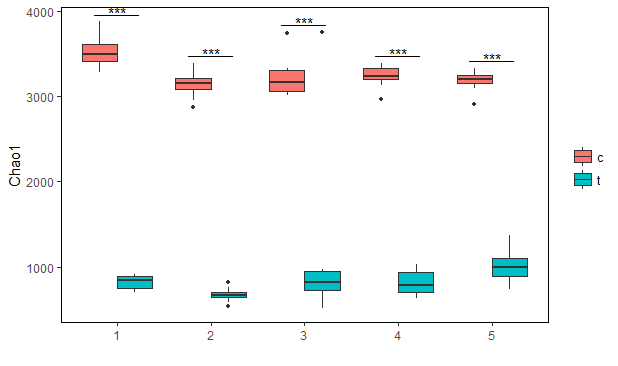#带显著性标记“abc”的箱线图， #先绘制箱线图主体 p geom_boxplot(outlier.size = 0.5, size = 0.5) + facet_wrap(~variable, 2, scales = 'free') + theme(panel.grid = element_blank(), panel.background = element_rect(fill = 'transparent', color = 'black'), legend.title = element_blank(), legend.key = element_blank()) + labs(x = '', y = 'Chao1') #再手动添加显著性标记 #同上所述，这里的显著性是提前通过差异分析已经计算好的，我们通过手动输入进来 alpha1_stat names(alpha1_stat) alpha1_stat$sig
p + geom_text(data = alpha1_stat, aes(label = sig, color = group1), position = position_dodge(1), vjust = -0.3)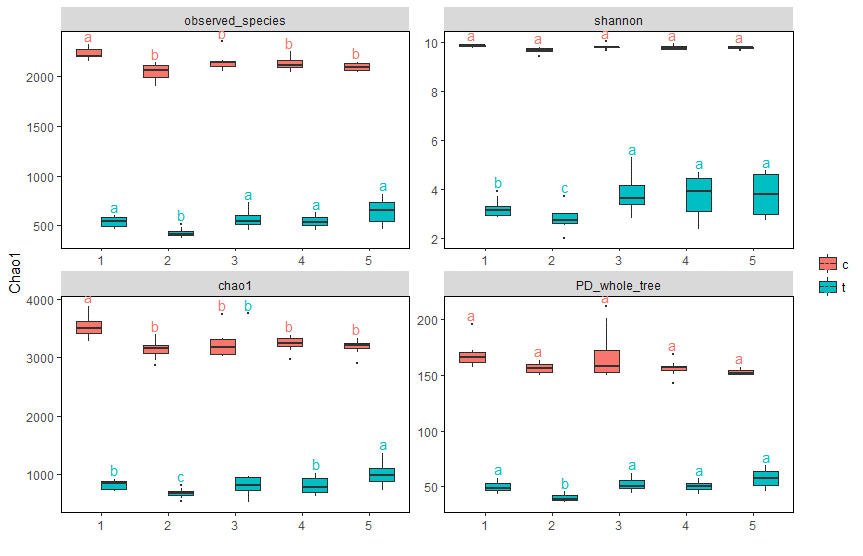好了，以上就是本篇的内容，大致简介了几种简单的箱线图在R中的绘制示例，以帮助刚接触R的同学们入门，get到了吗？友情链接R包vegan执行非参数多元方差分析(置换多元方差分析)

R包rcompanion执行非参数双因素方差分析(Scheirer-Ray-Hare检验)

R包sm执行非参数单因素协方差分析

R语言执行非参数单因素方差分析(Kruskal-Wallis检验、Friedman检验)

R语言执行多元方差分析

R语言执行重复测量方差分析

R语言执行双因素方差分析

R语言执行单因素协方差分析

R语言执行单因素方差分析及多重比较

R语言执行两组间差异分析Wilcoxon检验

R语言执行两组间差异分析T检验

R语言绘制分组柱状图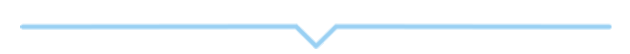展开全文• - apply(data3, 2,function(R) { ggplot(data) + aes(x = year, fill =R) + geom_bar(position = "fill") } ) #S3提取其中一幅ggplot图 p['age']$age #填入你循环画图的一个变量 #加标签 p2=p['age']$age+labs...r语言
• 要学习ggplot2，咱的思维得要大变，因为ggplot2无论是数据的输入格式还是作图的命令风格跟常规的R作图函数都有较大差别。1、首先ggplot2的输入格式就跟咱们常见的数据有点不一样假设我们要做个柱状图，常规R用的...
• r语言ggplot函数 Tidyverse包（例如ggplot2和dplyr）具有通常很方便的函数语法：您不必将列名放在引号中。 例如： dplyr::filter(mtcars, mpg > 30) 请注意，列名mpg未引用。 但是，如果要使用tidyverse编写...
• R语言ggplot中的颜色

千次阅读 2019-09-21 17:56:58
• R语言ggplot2修改坐标轴刻度

万次阅读 2020-01-04 15:26:43R语言 ggplot2 坐标轴刻度
• ggplot基础语句 折线图 ggplot(data,aes(x=,y=,colour=,group=))+ geom_line(size=1)+ labs(x='',y='',title='') + # 标题居中 theme(plot.title = element_text(hjust = 0.5)) 柱形图 ggplot(data,aes(x=,y=))...
• 写在前面，本文介绍的是grid包函数在ggplot2作图时的用处。由于要手动调整要素位置，要是你的需求是要业务化的作图，这篇文章可能没什么帮助。本次记录的是一个很神奇的需求，有3张数据来源完全不同，theme什么的一...
• 小伙伴们大家好，又到了积极教大家学习R语言可视化的时间了。所有的数据在最后呈现的时候都将以图象的形式直观的向大家展示，因此可视化可以说是科学数据处理的最后一步，所谓“编筐编篓,全在收口”，因此这最后一步...
• 写在前面，本文介绍的是grid包函数在ggplot2作图时的用处。由于要手动调整要素位置，要是你的需求是要业务化的作图，这篇文章可能没什么帮助。本次记录的是一个很神奇的需求，有3张数据来源完全不同，theme什么的一...
• R语言ggplot2可视化自定义图例（legend）示例点的大小实战 目录 R语言ggplot2可视化自定义图例（legend）示例点的大小实战 #ggplot2可视化自定义图例（legend）示例点的大小(size =1) #ggplot2可视化自定义...数据挖掘 机器学习 人工智能 自然语言处理 r语言
• geom_label()和ggplot_Text()的使用详解 主要是用于在图中标注文本 ??geom_label()可以查看其帮助文档 ##Examples p <- ggplot(mtcars, aes(wt, mpg, label = rownames(mtcars)))###建立一个新的画板，标记mtcars...
• R语言ggplot2-堆叠图

千次阅读 2021-04-28 09:29:24
如果你是电脑端，看到右下角的 “一键三连” 了吗，没错点它[哈哈] 要求 基本符合 head(diamonds) ggplot(data = diamonds)+ geom_bar(aes(x=cut,fill=clarity),position = "fill")+ coord_flip() 完美呈现 数据...R语言 ggplot2 堆叠图
• R语言ggplot2之图例的设置

千次阅读 2020-12-28 20:57:53
引言图例的设置包括移除图例、...library(ggplot2)p p + guides(fill=FALSE)123改变图例的位置我们可以用theme(legend.position=…)将图例移到图表的上方、下方、左边和右边。p scale_fill_brewer(palette="Pastel2......